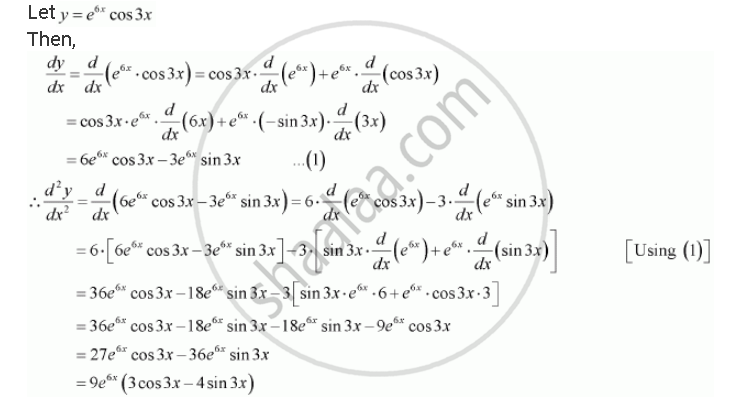Share

# Find the Second Order Derivatives of the Function. E6x Cos 3x - CBSE (Commerce) Class 12 - Mathematics

#### Question

Find the second order derivatives of the function.

e6x cos 3x

#### SolutionIs there an error in this question or solution?

#### APPEARS IN

NCERT Solution for Mathematics Textbook for Class 12 (2018 to Current)
Chapter 5: Continuity and Differentiability
Q: 7 | Page no. 183

#### Video TutorialsVIEW ALL 

Solution Find the Second Order Derivatives of the Function. E6x Cos 3x Concept: Second Order Derivative.
S